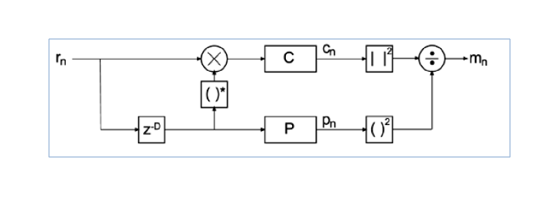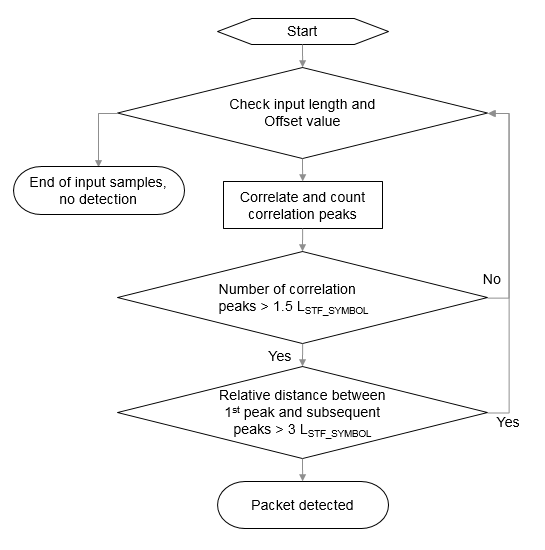# wlanPacketDetect

OFDM packet detection using L-STF

## Syntax

``startOffset = wlanPacketDetect(rxSig,cbw)``
``startOffset = wlanPacketDetect(rxSig,cbw,offset)``
``startOffset = wlanPacketDetect(rxSig,cbw,offset,threshold)``
``````[startOffset,M] = wlanPacketDetect(___)``````

## Description

example

````startOffset = wlanPacketDetect(rxSig,cbw)` returns the offset from the start of the input waveform to the start of the detected preamble, given a received time-domain waveform and the channel bandwidth. For more information, see Packet Detection Processing. NoteThis function supports packet detection of OFDM modulated signals only. ```

example

````startOffset = wlanPacketDetect(rxSig,cbw,offset)` specifies an offset from the start of the received waveform and indicates where the autocorrelation processing begins. The returned `startOffset` is relative to the input `offset`.```

example

````startOffset = wlanPacketDetect(rxSig,cbw,offset,threshold)` specifies the threshold which the decision statistic must meet or exceed to detect a packet. ```

example

``````[startOffset,M] = wlanPacketDetect(___)``` also returns the decision statistics of the packet detection algorithm for the received time-domain waveform, using any of the input arguments in the previous syntaxes.```

## Examples

collapse all

Detect a received 802.11n packet at a signal-to-noise ratio (SNR) of 20 dB.

Create an HT configuration object and TGn channel object. Generate a transmit waveform.

```cfgHT = wlanHTConfig; tgn = wlanTGnChannel('LargeScaleFadingEffect','None'); txWaveform = wlanWaveformGenerator([1;0;0;1],cfgHT);```

Pass the waveform through the TGn channel with an SNR of 20 dB. Detect the start of the packet.

```snr = 20; fadedSig = tgn(txWaveform); rxWaveform = awgn(fadedSig,snr,0); startOffset = wlanPacketDetect(rxWaveform,cfgHT.ChannelBandwidth)```
```startOffset = 1 ```

The packet is detected at the first sample of the received waveform, specifically the returned `startOffset` indicates an offset of zero samples from the start of the received waveform.

Detect a received 802.11ac packet that has been delayed. Specify an offset of 25 to begin the autocorrelation process.

Create an VHT configuration object and generate the transmit waveform.

```cfgVHT = wlanVHTConfig; txWaveform = wlanWaveformGenerator([1;0;0;1],cfgVHT,... 'WindowTransitionTime',0);```

Delay the signal by appending zeros at the start. Specify an offset of 25 for the beginning of autocorrelation processing. Detect the start of the packet.

```rxWaveform = [zeros(100,1);txWaveform]; offset = 25; startOffset = wlanPacketDetect(rxWaveform,cfgVHT.ChannelBandwidth,offset)```
```startOffset = 48 ```

Calculate the detected packet offset by adding the returned `startOffset` and the input `offset`.

`pktOffset = offset + startOffset`
```pktOffset = 73 ```

The offset from the first sample of the received waveform to the start of the packet is detected to be 73 samples. This coarse approximation of the packet-start offset is useful for determining where to begin autocorrelation for the first packet and for subsequent packets when a multipacket waveform is transmitted.

Detect a received 802.11a packet that has been delayed. No channel impairments are added. Set the input offset to 5 and use a threshold setting very close to 1.

Create an non-HT configuration object. Generate the transmit waveform.

```cfgNonHT = wlanNonHTConfig; txWaveform = wlanWaveformGenerator([1;0;0;1],cfgNonHT,... 'WindowTransitionTime',0);```

Delay the signal by appending zeros at the start. Set an initial offset of 5 and a threshold very close to 1. Detect the delayed packet.

```rxWaveform = [zeros(20,1);txWaveform]; offset = 5; threshold = 1-10*eps; startOffset = wlanPacketDetect(rxWaveform,... cfgNonHT.ChannelBandwidth,offset,threshold)```
```startOffset = 15 ```

Calculate the detected packet offset by adding the returned `startOffset` and the input `offset`.

`totalOffset = offset + startOffset`
```totalOffset = 20 ```

Using a threshold close to 1 and an undistorted received waveform increases the accuracy of the packet detect location. The detected offset from the first sample of the received waveform to the start of the packet is determined to be 20 samples.

Return the decision statistics of a WLAN waveform that consists of five 802.11a packets.

Create a non-HT configuration object and a five-packet waveform. Delay the waveform by 4000 samples.

```cfgNonHT = wlanNonHTConfig; txWaveform = wlanWaveformGenerator([1;0;0;1],cfgNonHT, ... 'NumPackets',5,'IdleTime',20e-6); rxWaveform = [zeros(4000,1);txWaveform];```

Setting the threshold input to 1, generates packet decision statistics for the entire waveform and suppresses the `startOffset` output. Plot the decision statistics, `M`.

```offset = 0; threshold = 1; [startOffset,M] = wlanPacketDetect(rxWaveform,cfgNonHT.ChannelBandwidth,... offset,threshold); plot(M)```Since `threshold` = 1, the decision statistics for the entire waveform are included in the output `M`. The decision statistics show five peaks. The peaks corresponds to the first sample of each packet detected. View `startOffset`.

`startOffset`
```startOffset = [] ```

The returned `startOffset` is empty because `threshold` was set to 1.

## Input Arguments

collapse all

Received time-domain signal, specified as an NS-by-NR matrix. NR is the number of receive antennas. NS represents the number of time-domain samples in the received signal.

Data Types: `double`
Complex Number Support: Yes

Channel bandwidth in MHz, specified as `'CBW5'`, `'CBW10'`, `'CBW20'`, `'CBW40'`, `'CBW80'`, or `'CBW160'`.

Data Types: `char` | `string`

Number of samples offset from the beginning of the received waveform, specified as a nonnegative integer. `offset` defines the starting sample for the autocorrelation process. `offset` is useful for advancing through and detecting the `startOffset` sample for successive packets in multipacket waveforms.

Note

Since the packet detection searches forward in time, the first packet will not be detected if the initial setting for `offset` is beyond the first L-STF.

Data Types: `double`

Decision statistic threshold that must be met or exceeded to detect a packet, specified as a real scalar greater than 0 and less than or equal to 1.

Data Types: `double`

## Output Arguments

collapse all

Number of samples offset to the start of packet, returned as a nonnegative integer. This value, shifted by `offset`, indicates the detected start of a packet from the first sample of `rxSig`.

• An empty value, `[ ]`, is returned if no packet is detected or if `threshold` is set to 1.

• Zero is returned if there is no delay, specifically the packet is detected at the first sample of the waveform.

Decision statistics based on autocorrelation of the input waveform, returned as an N-by-1 real vector. The length of N depends on the starting location of the autocorrelation process and the number of samples until a packet is detected. When `threshold` is set to 1, `M` returns the decision statistics of the full waveform and `startOffset` returns empty.

collapse all

### L-STF

The legacy short training field (L-STF) is the first field of the 802.11™ OFDM PLCP legacy preamble. The L-STF is a component of VHT, HT, and non-HT PPDUs.The L-STF duration varies with channel bandwidth.

Channel Bandwidth (MHz)Subcarrier Frequency Spacing, ΔF (kHz)Fast Fourier Transform (FFT) Period (TFFT = 1 / ΔF)L-STF Duration (TSHORT = 10 × TFFT / 4)
20, 40, 80, and 160312.53.2 μs8 μs
10156.256.4 μs16 μs
578.12512.8 μs32 μs

Because the sequence has good correlation properties, it is used for start-of-packet detection, for coarse frequency correction, and for setting the AGC. The sequence uses 12 of the 52 subcarriers that are available per 20 MHz channel bandwidth segment. For 5 MHz, 10 MHz, and 20 MHz bandwidths, the number of channel bandwidths segments is 1.

## Algorithms

collapse all

### Packet Detection Processing

The packet detection algorithm is implemented as a double sliding window as described in OFDM Wireless LANs , Chapter 2. The autocorrelation of L-STF short training symbols is used to return an estimated packet-start offset. In a robust system, the next stage will refine this estimate with symbol timing detection using the L-LTF.

As shown in the figure, the received signal, rn, is delayed then correlated in two sliding windows independently. The packet detection processing output provides decision statistics (mn) of the received waveform.• Window C autocorrelates between the received signal and the delayed version, cn.

`${c}_{n}=\sum _{l=1}^{{N}_{R}}\sum _{K=0}^{D-1}{r}_{n+k,l}{r}_{n+k+D,l}^{*}$`

• Window P calculates the energy received in the autocorrelation window, pn.

`${p}_{n}=\sum _{l=1}^{{N}_{R}}\sum _{k=0}^{D-1}|{r}_{n+k+D,l}{|}^{2}$`

• The decision statistics, mn, normalize the autocorrelation by pn so that the decision statistic is not dependent on the absolute received power level.

`${m}_{n}=\frac{|{c}_{n}{|}^{2}}{{\left({p}_{n}\right)}^{2}}$`

The decision statistics provide visual information resulting from the autocorrelation process that is useful when selecting the appropriate threshold value for the input waveform. The recommended default value of 0.5 for `threshold` favors false detections over missed detections considering a range of SNRs and various antenna configurations.

In the sliding window calculations, D is the period of the L-STF short training symbols and NR is the number of receive antennas.

Packet detection processing follows this flow chart:LSTF_SYMBOL is the length of an L-STF symbol.

Note

This function supports packet detection of OFDM modulated signals only.

 Terry, J., and J. Heiskala. OFDM Wireless LANs: A Theoretical and Practical Guide. Indianapolis, IN: Sams, 2002.

## SupportGet trial now